### IMO Shortlist 2009 problem G1

Kvaliteta:
Avg: 0,0
Težina:
Avg: 6,0
Let$ABC$ be a triangle with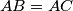$AB = AC$ . The angle bisectors of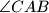$\angle C AB$ and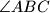$\angle AB C$ meet the sides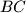$B C$ and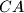$C A$ at$D$ and$E$ , respectively. Let$K$ be the incentre of triangle$ADC$. Suppose that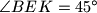$\angle B E K = 45^\circ$ . Find all possible values of$\angle C AB$ .

Jan Vonk, Belgium, Peter Vandendriessche, Belgium and Hojoo Lee, Korea
Izvor: Međunarodna matematička olimpijada, shortlist 2009